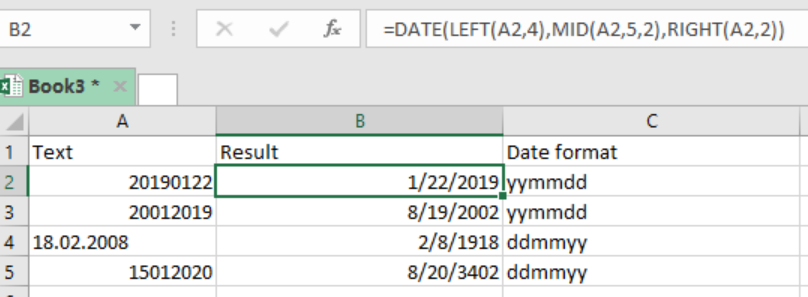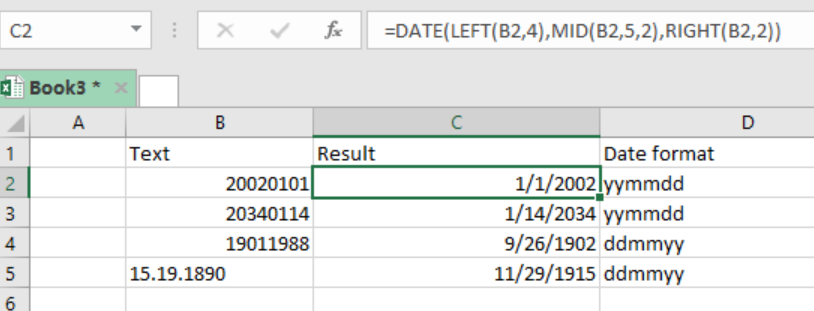Get instant live expert help with Excel or Google Sheets“My Excelchat expert helped me in less than 20 minutes, saving me what would have been 5 hours of work!”

#### Post your problem and you'll get expert help in seconds

Your message must be at least 40 characters
Our professional experts are available now. Your privacy is guaranteed.

# Convert text to date

In excel, it is possible to convert text which is in an unrecognized format into a proper excel date. We can do this by parsing the text and assembling a proper date using a formula that is based on functions like; DATE, LEFT, MID and RIGHT. This article provides a conclusive procedure on how to convert text to date.Figure 1: Converting text to date in Excel

## General syntax of the formula

`=DATE(LEFT(text, 4), MID(text,5,2), RIGHT(text, 2)`

## Understanding the formula

The formula above is fundamental when we want to convert a give text which is in an unrecognized format into an Excel recognized format. This procedure is most crucial especially when you are working with text from another system, which might arrange the dates in a different format other than that recognized by excel.

## How the formula works

• DATE function is composed of three arguments, the year, month and day arguments.
• In the formula, we have the LEFT function, which is tasked to extract the 4 leftmost characters and then supplies them to the DATE as year.
• We also have the MID function which is responsible for extracting the characters 5-6 and then supplies them to the DATE function as month.
• The RIGHT function will extract the 2 rightmost characters and supply them to the DATE function as day.
• These then will be arranged in a format that can easily be recognized by excel as dates.

## Fixing dates by adding zero

There are times when we get dates in text formats recognizable by excel. Here, we can force Excel to convert these texts into dates by simply adding zero to the given value.

Excel coerces text values into numbers when a zero is added.

For you to fix dates by adding zero, follow the following procedure;

• Select an unused cell and add a zero in it, then copy it to the clipboard.
• Select the dates that you need to convert

You can also do this by using the formula below;

`=A1 + 0`

## ExampleFigure 2: Example of how to convert text to date

• In this example, we want to find the correct date format of the date texts in column B.
• To do this, we need to use the formula as stated above to force excel convert the texts into recognizable date format.
• We shall enter our formula, =DATE(LEFT(B2,4),MID(B2,5,2),RIGHT(B2,2)),  to cell C2.
• Then, we Press the Enter key to get the date format for that cell.
• We then copy down the formula across the other cells to get their correct date format.

## Instant Connection to an Expert through our Excelchat Service

Most of the time, the problem you will need to solve will be more complex than a simple application of a formula or function. If you want to save hours of research and frustration, try our live Excelchat service! Our Excel Experts are available 24/7 to answer any Excel question you may have. We guarantee a connection within 30 seconds and a customized solution within 20 minutes.

### Did this post not answer your question? Get a solution from connecting with the expert.Another blog reader asked this question today on Excelchat:## Subscribe to Excelchat.coAnother blog reader asked this question today on Excelchat: